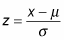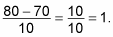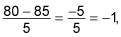##### Statistics For DummiesIf you have a statistical sample with a normal distribution, you can plug an x-value for this distribution into a special equation to find its z-value. The z-value can then help you to interpret statistical values such as finding out whether a student's relative standing is better in one class than another.

Changing an x-value to a z-value is called standardizing. The so-called "z-formula" for standardizing an x-value to a z-value is:You take your x-value, subtract the mean , and then divide this difference by the standard deviation. This gives you the corresponding standard score (z-value or z-score).

Standardizing is just like changing units (for example, from Fahrenheit to Celsius). It doesn't affect probabilities for X.

You can standardize an x-value from any distribution (not just the normal) using the z-formula. Similarly, not all standard scores come from a normal distribution.

Because you subtract the mean from your x-values and divide everything by the standard deviation when you standardize, you are literally taking the mean and standard deviation out of the equation. This is what allows you to compare everything on the Z-distribution where negative values indicate being below the mean, positive values indicate being above the mean, and a value of 0 indicates you're right on the mean.

Standardizing also allows you to compare numbers from different distributions. For example, suppose Bob scores 80 on both his math exam (which has a mean of 70 and standard deviation of 10) and his English exam (which has a mean of 85 and standard deviation of 5). On which exam did Bob do better, in terms of his relative standing in the class?

Bob's math exam score of 80 standardizes to a z-value ofThat tells us his math score is one standard deviation above the class average. His English exam score of 80 standardizes to a z-value ofputting him one standard deviation below the class average. Even though Bob scored 80 on both exams, he actually did better on the math exam than the English exam, relatively speaking.

To interpret a standard score, you don't need to know the original score, the mean, or the standard deviation. The standard score gives you the relative standing of a value, which in most cases is what matters most. In fact, on most national achievement tests, they won't even tell you what the mean and standard deviation were when they report your results; they just tell you where you stand on the distribution by giving you your z-score.